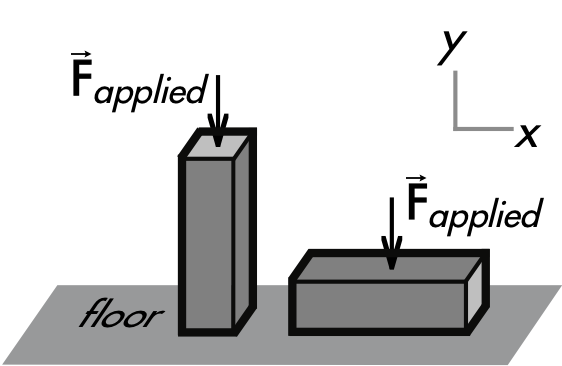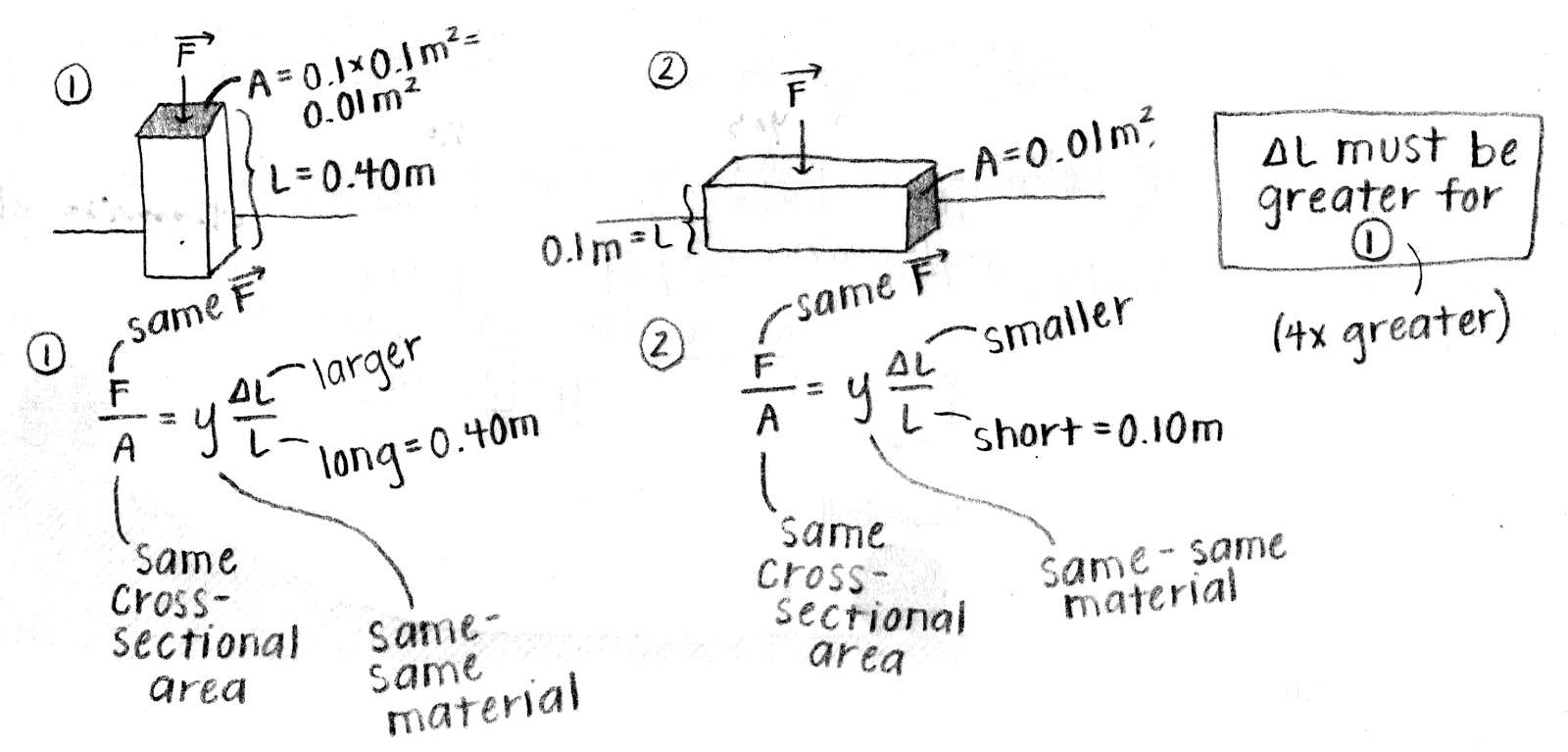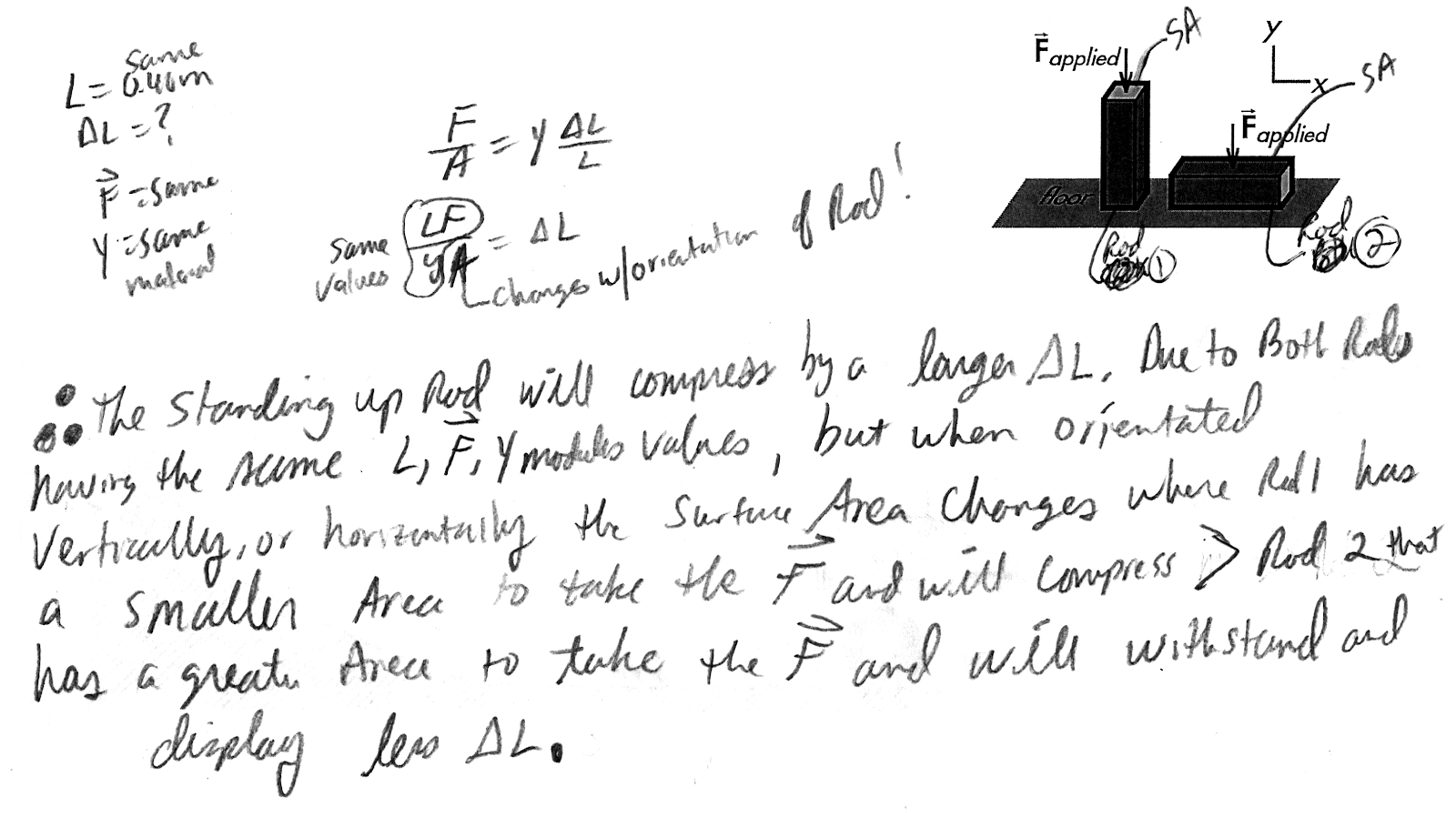## 20191123

### Physics midterm question: comparing compression of rod in different orientations

Physics 205A Midterm 2, fall semester 2019
Cuesta College, San Luis Obispo, CAA 0.40 m long copper rod with a square profile (0.10 m × 0.10 m) can be oriented standing up, or laid down on its side on a floor. If the same amount of downwards force is applied to the top surface in each case, discuss whether the standing-up rod or the laid-down rod will compress a greater ∆L amount (or if there will be a tie). Explain your reasoning using the properties of stress, strain, and Hooke's law.

• p:
Correct. Applies Hooke's law in a systematic manner by:
1. recognizing that the applied force F and Young's modulus Y are the same for both rods; and
2. as a result ΔL depends only on the original length L divided by cross-sectional area A; and
3. since the standing-up rod has a longer original length (L = 0.40 m) and a smaller cross-sectional area (A = 0.010 m2), it will compress more than the laid-down rod with a shorter original length (L = 0.10 m) and a greater cross-sectional area (A = 0.040 m2).
• r:
As (p), but argument indirectly, weakly, or only by definition supports the statement to be proven, or has minor inconsistencies or loopholes.
• t:
Nearly correct, but argument has conceptual errors, or is incomplete. Considers only difference in cross-sectional areas (neglecting the difference in original lengths), or vice versa; or recognizes both differences but somehow argues that the rods will still compress by the same amount.
• v:
Limited relevant discussion of supporting evidence of at least some merit, but in an inconsistent or unclear manner. At least some systematic attempt at using Hooke's law quantities.
• x:
Implementation/application of ideas, but credit given for effort rather than merit. Approach other than that of relating strain (force per unit area), Young's modulus, and strain using Hooke's law.
• y:
Irrelevant discussion/effectively blank.
• z:
Blank.
Sections 70854, 70855
Exam code: midterm02sQm5
p: 18 students
r: 1 student
t: 28 students
v: 3 students
x: 2 students
y: 0 students
z: 0 students

A sample "p" response (from student 2586):A sample "t" response (from student 6672), recognizing that the original length L changes with different orientation, but claims the cross-sectional area A is the same:A sample "t" response (from student 2875), recognizing that the cross-sectional area A changes with different orientation, but claims the original length L is the same: